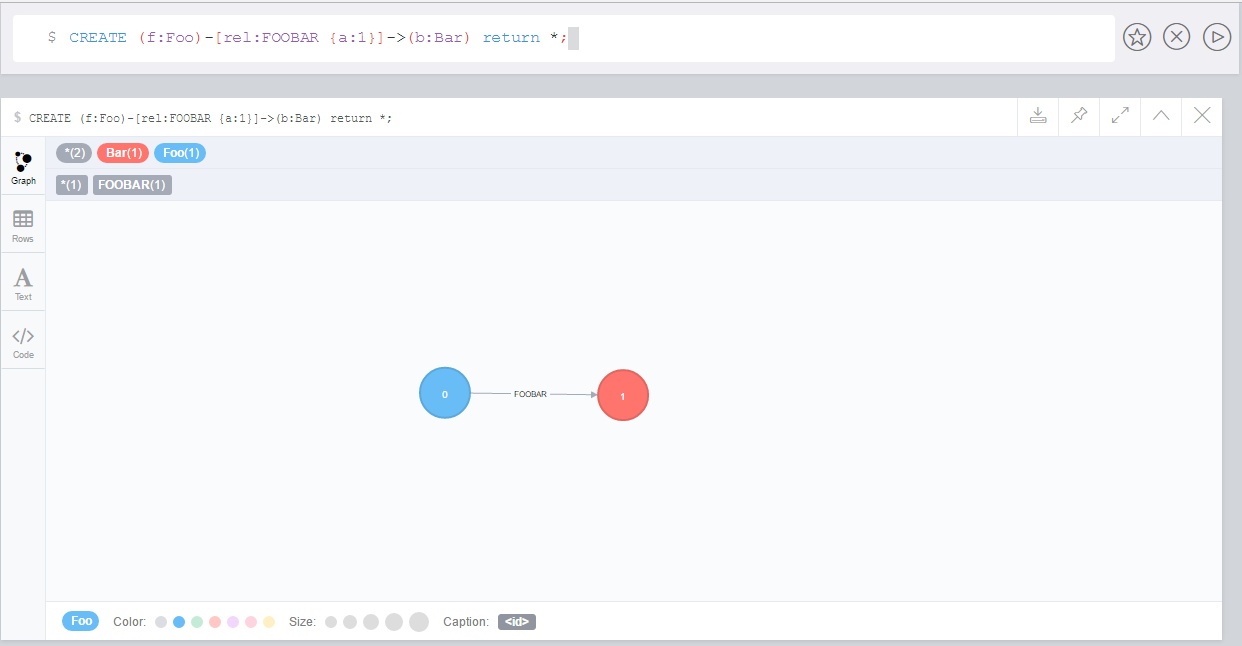# Invert relationship

The available procedure is described in the table below:

 `call apoc.refactor.invert(rel)` inverts relationship direction

## Example Usage

The example below will help us learn how to use this procedure.

The following creates a graph containing two nodes connected by a relationship:
``CREATE (f:Foo)-[rel:FOOBAR {a:1}]->(b:Bar)``The following inverts the direction of the relationship:
``````MATCH (f:Foo)-[rel:FOOBAR {a:1}]->(b:Bar)
CALL apoc.refactor.invert(rel)
yield input, output
RETURN input, output``````

If we execute this query, it will result in the following graph: